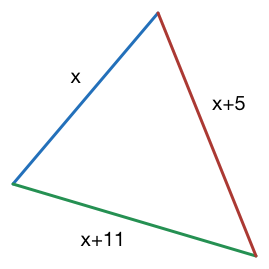Algebra

# Triangle Inequality

The side lengths of a non-degenerate $\triangle ABC$ are $4, 11$ and $c.$ Which of the following is a possible value for $c?$

Two legs of a triangle have lengths of 7.4 and 17.3 respectively. Given that the length of the third side is a whole number, what is the largest possible length for the third side?The three side lengths of a non-degenerate triangle are $x, x + 5$ and $x + 11.$ Which of the following is NOT a possible value for $x?$

Three positive integers $a, b$ and $c$ are the side lengths of $\triangle ABC.$ If $c$ is the longest side and $a + b + c = 21,$ what is the maximum value of $c?$

Two of the legs of an isosceles triangle have length 12 and 27 respectively. What is the perimeter of the triangle?

Details and assumptions

An isosceles triangle is a triangle with exactly two equal sides.

×